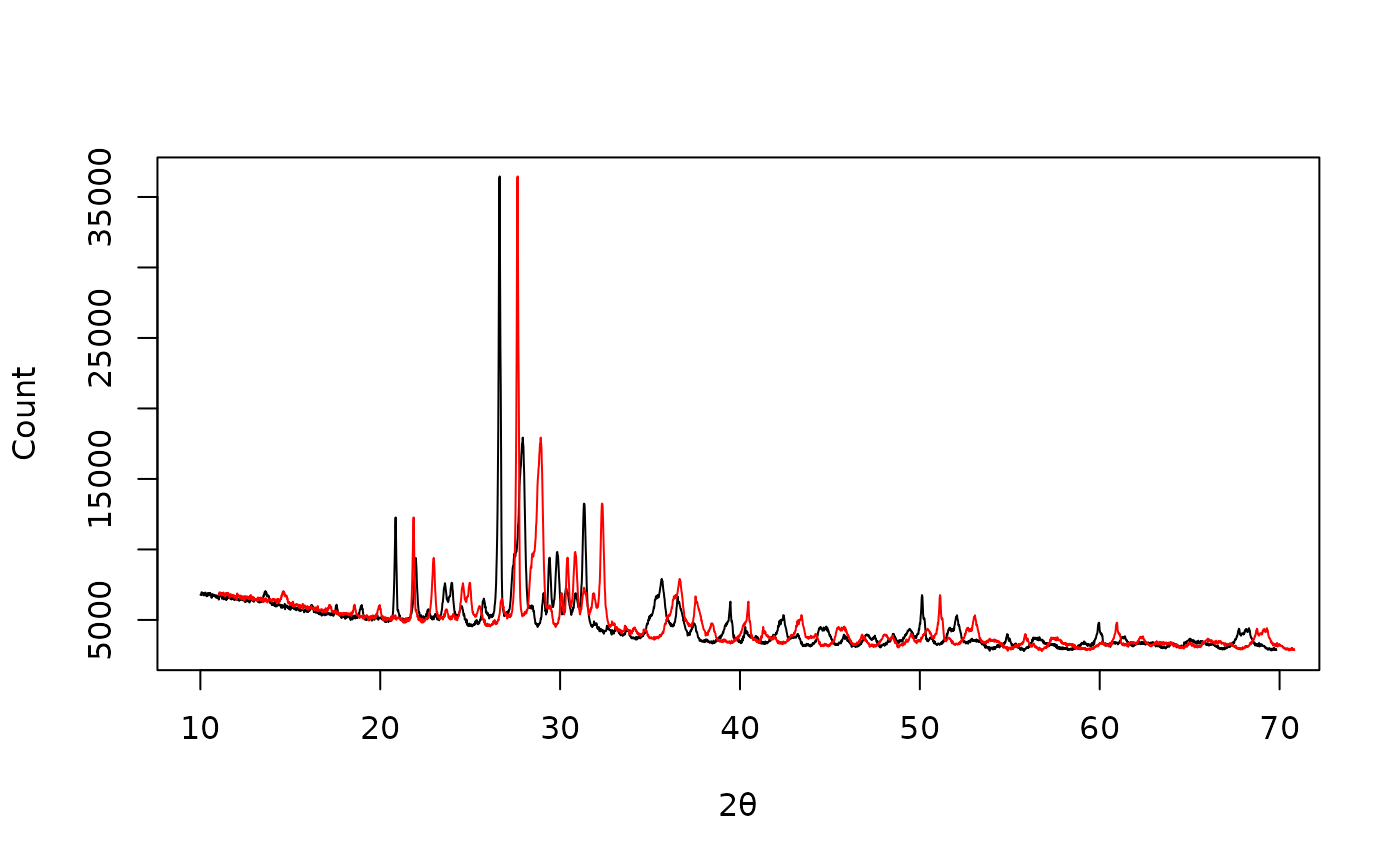Shifts the x scale by a given value.

## Usage

signal_shift(x, y, lag, ...)

# S4 method for numeric,numeric
signal_shift(x, y, lag)

# S4 method for ANY,missing
signal_shift(x, lag)

## Arguments

x, y

A numeric vector. If y is missing, an attempt is made to interpret x in a suitable way (see grDevices::xy.coords()).

lag

A numeric vector specifying the offset.

...

Currently not used.

## Value

Returns a list with two components x and y.

Other signal processing methods: signal_bind(), signal_correct(), signal_drift(), signal_mean(), subset()

N. Frerebeau

## Examples

## X-ray diffraction
data("XRD")

## Plot spectrum
plot(XRD, type = "l", xlab = expression(2*theta), ylab = "Count")

## Shift by one degree
XRD_offset <- signal_shift(XRD, lag = 1)
lines(XRD_offset, type = "l", col = "red")